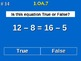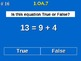# 1st Grade Common Core Math - Determine If Equations Are True Or False 1.OA.7Subject
Resource Type
Product Rating
File Type

Presentation (Powerpoint) File

Be sure that you have an application to open this file type before downloading and/or purchasing.

412 KB|129 pages
Share
Product Description
First Grade Common Core Math - Determine If Equations Involving Addition & Subtraction Are True Or False 1.OA.7 Practice provides two ways for students to practice and show mastery of their ability to determine if equations involving addition and subtraction are true or false.

It includes 40 distinct problems in two sets (129 slides in all!), with and without following answer slides. The PowerPoint file can be used on computers, or Promethean Activboard and Smart boards.

Take a look at the preview file and buy today for your students benefit!

Standard 1 OA.7 Work with addition and subtraction equations.

7. Understand the meaning of the equal sign, and determine if equations involving addition and subtraction are true or false. For example, which of the following equations are true and which are false? 6 = 6, 7 = 8 – 1, 5 + 2 = 2 + 5, 4 + 1 = 5 + 2.
Total Pages
129 pages